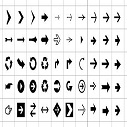# Array in Python

An array data structure belongs to the “must-import” category. To use an array in Python, you’ll need to import this data structure from the NumPy package or the array module.

Python has built-in data structures like lists, tuples, sets, and dictionaries for which there is no need to include any package or module.

An array is a data structure that stores a collection of items. Like lists, arrays are ordered, mutable, enclosed in square brackets, and able to store non-unique items.

But when it comes to the array’s ability to store different data types, the answer is not as straightforward. It depends on the kind of array used.

To use arrays in Python, you need to import either an array module or a NumPy package.

The Python array module requires all array elements to be of the same type. Moreover, to create an array, you’ll need to specify a value type. In the code below, the “i” signifies that all elements in array_1 are integers:

```import array as arr

array_1 = arr.array("i", [3, 6, 9, 12])
print(array_1)
print(type(array_1))
```

### Output:

array('i', [3, 6, 9, 12])
<class 'array.array'>

On the other hand, NumPy arrays support different data types. To create a NumPy array, you only need to specify the items (enclosed in square brackets, of course):

```import numpy as np

array_2 = np.array(["numbers", 3, 6, 9, 12])
print (array_2)
print(type(array_2))
```

### Output:

['numbers' '3' '6' '9' '12']
<class 'numpy.ndarray'>

As you can see, array_2 contains one item of the string type (i.e., “numbers”) and four integers.

### Differences

• Arrays need to be declared. Lists don’t, since they are built into Python. In the examples above, you saw that lists are created by simply enclosing a sequence of elements into square brackets. Creating an array, on the other hand, requires a specific function from either the array module (i.e., array.array()) or NumPy package (i.e., numpy.array()). Because of this, lists are used more often than arrays.
• Arrays can store data very compactly and are more efficient for storing large amounts of data.
• Arrays are great for numerical operations; lists cannot directly handle math operations. For example, you can divide each element of an array by the same number with just one line of code. If you try the same with a list, you’ll get an error.
```array = np.array([3, 6, 9, 12])
division = array/3
print(division)
print (type(division))
```

### Output:

[1. 2. 3. 4.] <class 'numpy.ndarray'>
```list = [3, 6, 9, 12]
division = list/3
```

### Output:

TypeError Traceback (most recent call last)
in
1 list = [3, 6, 9, 12]
----> 2 division = list/3

TypeError: unsupported operand type(s) for /: 'list' and 'int'

Of course, it’s possible to do a mathematical operation with a list, but it’s much less efficient

### When should you use a list and when should you use an array

• If you need to store a relatively short sequence of items and you don’t plan to do any mathematical operations with it, a list is the preferred choice. This data structure will allow you to store an ordered, mutable, and indexed sequence of items without importing any additional modules or packages.
• If you have a very long sequence of items, consider using an array. This structure offers more efficient data storage.
• If you plan to do any numerical operations with your combination of items, use an array. Data analytics and data science rely heavily on (mostly NumPy) arrays.Uk01 on Jun 22, 2020 at 12:05 am

If you like dEexams.com and would like to contribute, you can write your article here or mail your article to admin@deexams.com . See your article appearing on the dEexams.com main page and help others to learn.

#### Post Comment

##### WEB TECHNOLOGY##### TypeScript Programmning Language##### New HTML5 APIs##### HTML Arrow -> Range: Decimal 8592-8703. Hex 2190-21FF.##### How To Override One CSS Class With Another×